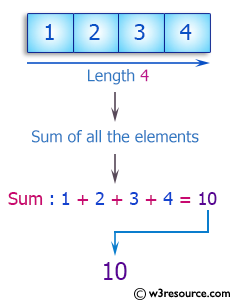﻿ Swift Array Programming Exercise: Compute the sum of all the elements of a given an array of integers and length 4 - w3resource# Swift Array Programming Exercises: Compute the sum of all the elements of a given an array of integers and length 4

## Swift Array Programming: Exercise-4 with Solution

Write a Swift program to compute the sum of all the elements of a given an array of integers and length 4.

Pictorial Presentation:Sample Solution:

Swift Code:

``````func sum4(_ arra: [Int]) -> Int {
return arra.reduce(0, +)
}
print(sum4([1, 2, 3, 4]))
print(sum4([1, 5, 7, 11]))
print(sum4([-5, 4, 1, 0]))
```
```

Sample Output:

```10
24
0
```

Swift Programming Code Editor:

Improve this sample solution and post your code through Disqus

What is the difficulty level of this exercise?

﻿